# Multivariate views#

In this notebook, we show a few examples of how to have plots with graphs of different types in a figure, like having a scatter plot with marginal distributions or even a multivariate plot with pair relationships of all properties in a table.

Because these plots involve managing subplots, they are all figure-level functions.

import pandas as pd
import numpy as np
import matplotlib.pyplot as plt
import seaborn as sns


We start by loading a table of measurments into a pandas DataFrame.

df = pd.read_csv("../../data/BBBC007_analysis.csv")
df

area intensity_mean major_axis_length minor_axis_length aspect_ratio file_name
0 139 96.546763 17.504104 10.292770 1.700621 20P1_POS0010_D_1UL
1 360 86.613889 35.746808 14.983124 2.385805 20P1_POS0010_D_1UL
2 43 91.488372 12.967884 4.351573 2.980045 20P1_POS0010_D_1UL
3 140 73.742857 18.940508 10.314404 1.836316 20P1_POS0010_D_1UL
4 144 89.375000 13.639308 13.458532 1.013432 20P1_POS0010_D_1UL
... ... ... ... ... ... ...
106 305 88.252459 20.226532 19.244210 1.051045 20P1_POS0007_D_1UL
107 593 89.905565 36.508370 21.365394 1.708762 20P1_POS0007_D_1UL
108 289 106.851211 20.427809 18.221452 1.121086 20P1_POS0007_D_1UL
109 277 100.664260 20.307965 17.432920 1.164920 20P1_POS0007_D_1UL
110 46 70.869565 11.648895 5.298003 2.198733 20P1_POS0007_D_1UL

111 rows × 6 columns

## Plotting joint and marginal distributions#

To have a joint distribution of two variables with the marginal distributions on the sides, we can use jointplot.

sns.jointplot(data=df, x="aspect_ratio", y="area")

<seaborn.axisgrid.JointGrid at 0x250479ad070>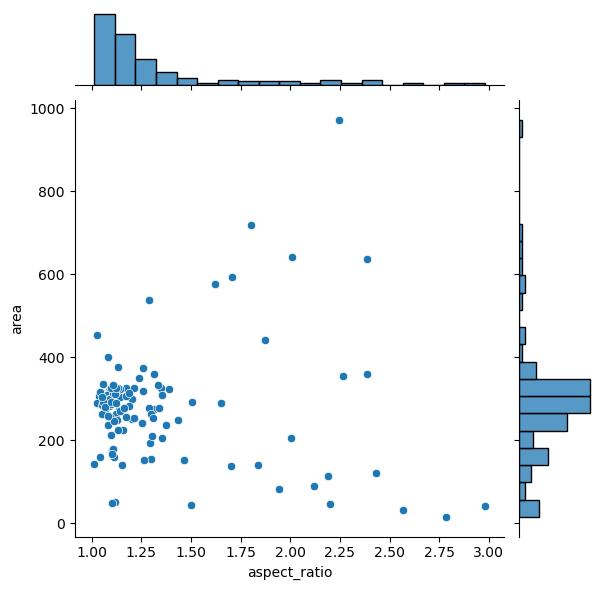It is possible to separate groups by passing a categorical property to the hue argument. This has an effect on the marginal distribution, turning them from histogram to kde plots.

sns.jointplot(data=df, x="aspect_ratio", y="area", hue = 'file_name')

<seaborn.axisgrid.JointGrid at 0x250479c9d90>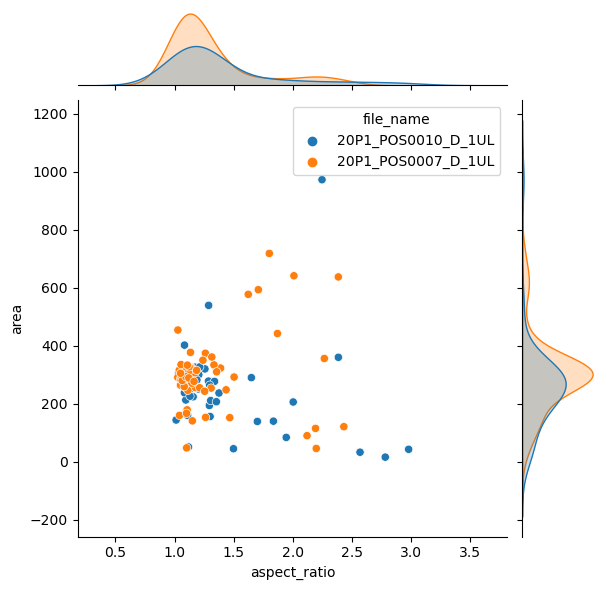## Plotting many distributions at once#

The above examples displayed a plot with relationship between two properties. This can be further expanded with the pairplot function

sns.pairplot(data=df)

<seaborn.axisgrid.PairGrid at 0x2504805e730>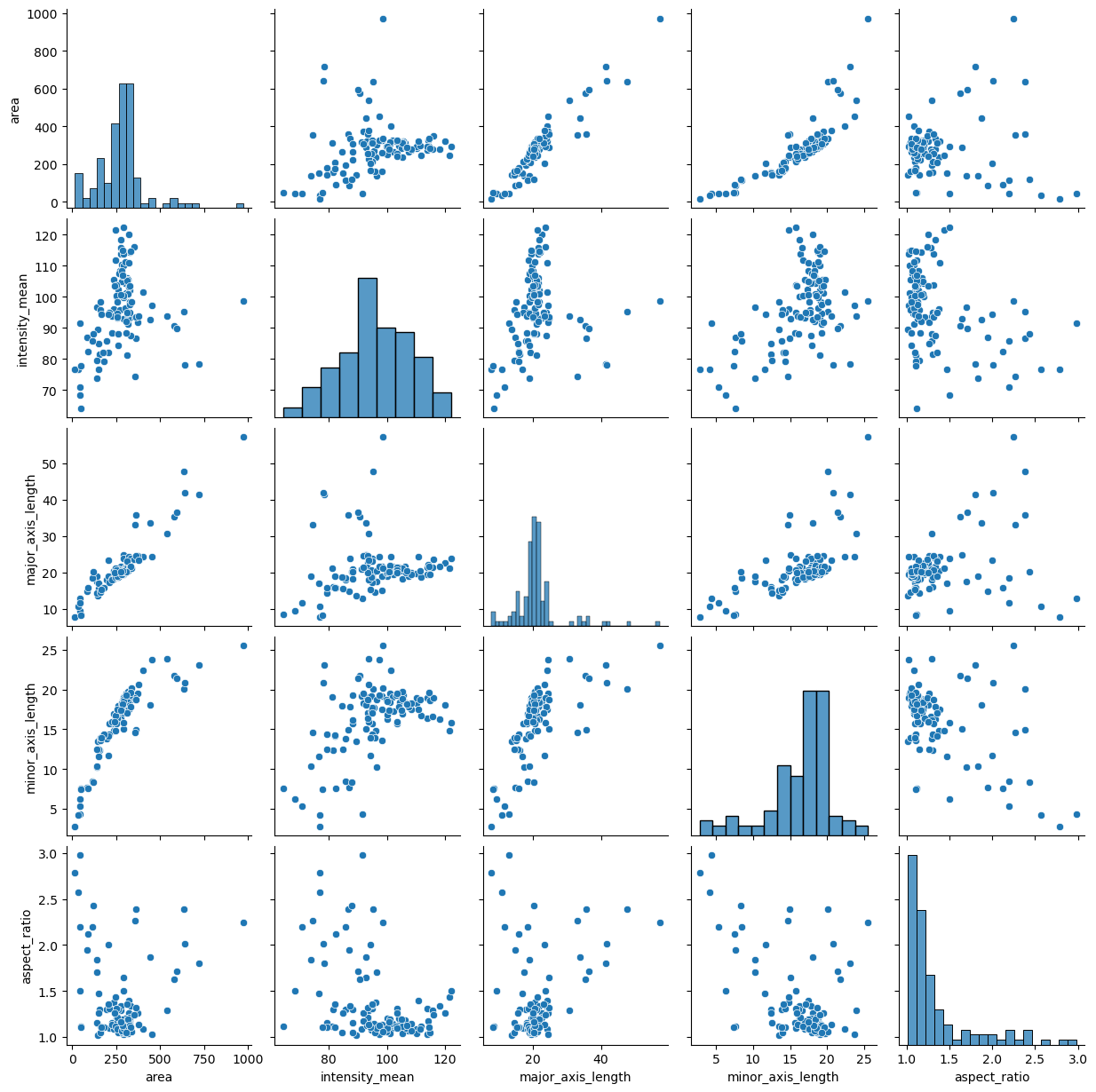sns.pairplot(data=df, hue="file_name")

<seaborn.axisgrid.PairGrid at 0x2504a5fba60>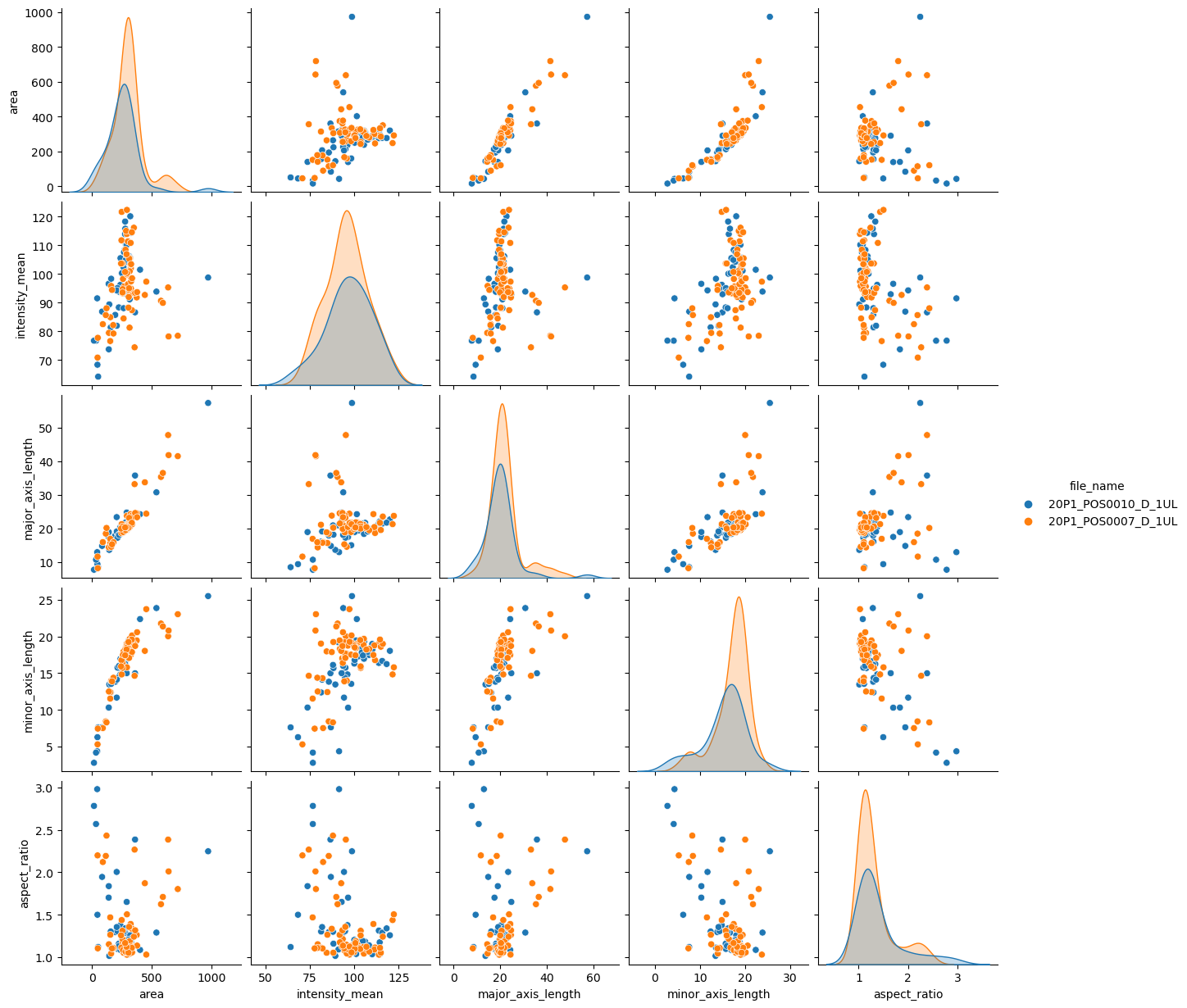If you have too many points, displaying every single point may yield graphs too poluted. An alternative visualization in this case could be a 2D histogram plot. We can do that by changing the kind argument to “hist”.

sns.pairplot(data=df, hue="file_name", kind = "hist")

<seaborn.axisgrid.PairGrid at 0x2504a613e50>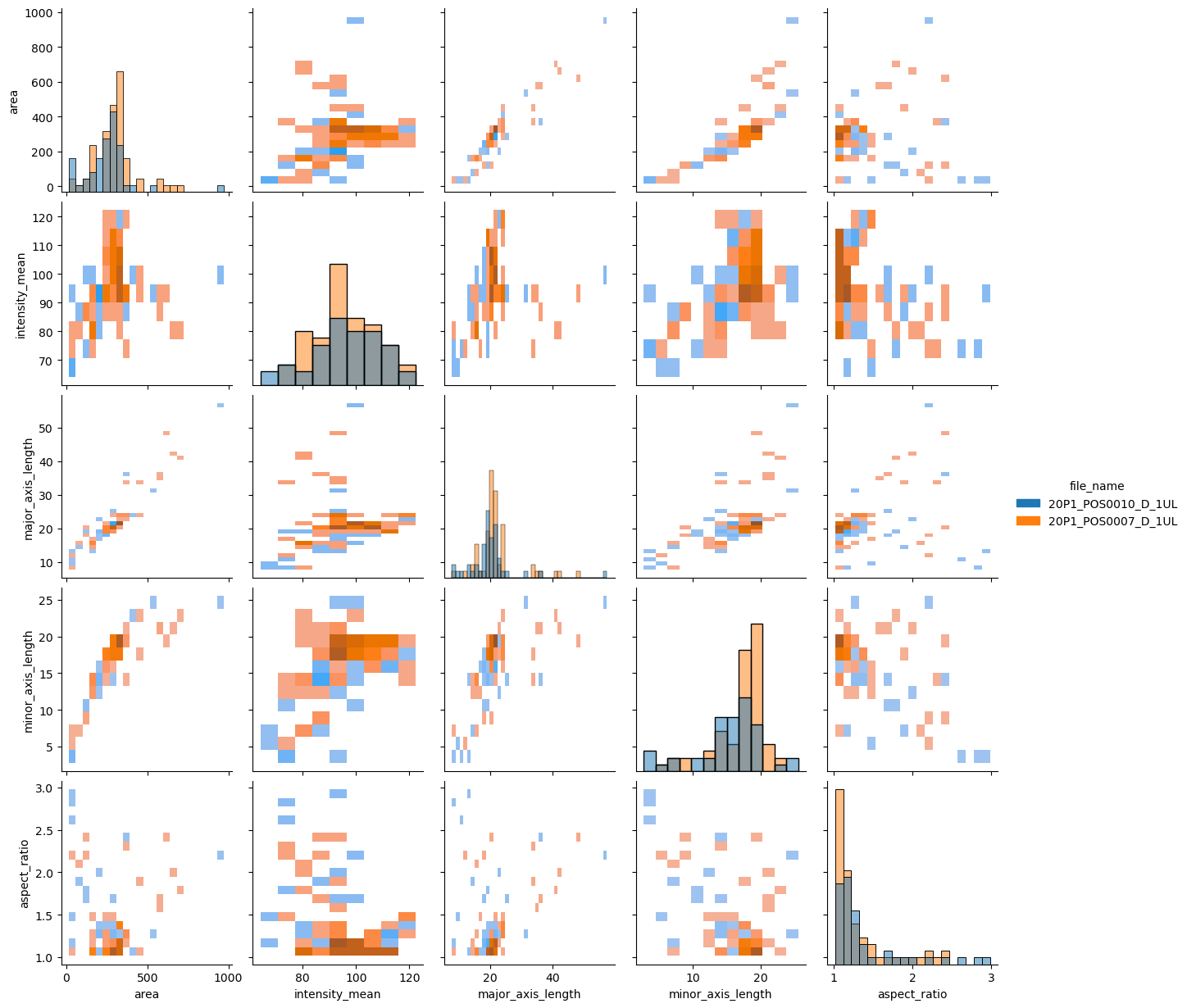## Exercise#

You may have noticed that the pairplot is redundant in some plots because the upper diagonal displays the same relationships rotated.

Redraw the pairplot to display only the lower diagonal of the plots.

Hint: explore the properties of the pairplot